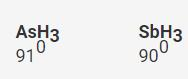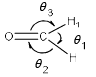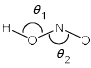Courses

# Test: Chemical Bonding- 3

## 30 Questions MCQ Test Inorganic Chemistry | Test: Chemical Bonding- 3

Description
This mock test of Test: Chemical Bonding- 3 for Chemistry helps you for every Chemistry entrance exam. This contains 30 Multiple Choice Questions for Chemistry Test: Chemical Bonding- 3 (mcq) to study with solutions a complete question bank. The solved questions answers in this Test: Chemical Bonding- 3 quiz give you a good mix of easy questions and tough questions. Chemistry students definitely take this Test: Chemical Bonding- 3 exercise for a better result in the exam. You can find other Test: Chemical Bonding- 3 extra questions, long questions & short questions for Chemistry on EduRev as well by searching above.
QUESTION: 1

Solution:
QUESTION: 2

Solution:
QUESTION: 3

### The correct non-linear and iso-structural pair is:

Solution:

In B the central atoms are S and I+ respectively, having 6 e- each and the others are from G-VII. Hence, they will have the same lone pair repulsion.

Hence B is correct.

QUESTION: 4

Among the following pairs, those in which both the species have the similar shape:

(I) N3-, XeF2
(II) [ClF2]+, [ICl2]+
(III) [ICl4]+, [PtCl4]2-
(IV) XeO3, SO3

Solution:
QUESTION: 5

According to VSEPR theory, the molecule/ ion having ideal tetrahedral shape is:

Solution:
QUESTION: 6

Which among are super valent species:

(I) NO
(II) NO2
(III) PCl5
(IV) SF6

Solution:
QUESTION: 7

Correct order of bond angles is:

Solution:Electronegativity decreases down the group, the electron density also decreases and consequently the repulsive interactions between the electron pairs also decrease thereby decreasing the bond angle H-M-H

QUESTION: 8

The compound pair which shows correct order of angle:

(I) NH3 > PH3
(II) H2S < H2O
(III) NO2+ > NO2
(IV) NO2+ > NO2
(V) NO2 > NO2+

Solution:
QUESTION: 9

The bond energies of H2, F2 and Cl2 decreases in the following order:

Solution:
QUESTION: 10

Arrange the following molecules in order of increasing bond polarit :

(I) H2O
(II) NH3
(III) PH3
(IV) H2S

Solution:
QUESTION: 11

The element NaCl and Kr crystallizes in a cubic structure. While NaCl is a solid at room temperature, Kr is a gas? The reason for this is:

Solution:
QUESTION: 12

Which of the following molecules will have a permanent dipole moment?

Solution:
QUESTION: 13

The molecules NO2 and N2O4 are respectively:

Solution:
QUESTION: 14

The molecule which has a zero dipole moment is:

Solution:
QUESTION: 15

Ion-dipole interactions are:

Solution:
QUESTION: 16

Which is/are paramagnetic among:

(I) S2 gas
(II) O2
(III) NO2+
(IV) CaH2

Solution:
QUESTION: 17

Which has the tendency to form covalent compound?

Solution:
QUESTION: 18

The molecule which show back donation is:

Solution:
QUESTION: 19

P2 coverts into P4 due to:

Solution:
QUESTION: 20

Which has an exact angle of 120° among:

Solution:
QUESTION: 21

The correct order of bond angles θ1, θ2 and θ3 in the following isSolution:
QUESTION: 22

Correct order of bond angle is:

Solution:
QUESTION: 23

Correct order of bond angle is:

Solution:
QUESTION: 24

Which of the following is correct:Solution:
QUESTION: 25

Which of the following does not exist:

Solution:
QUESTION: 26

Which of the following is most polar bond:

Solution:
QUESTION: 27

Which will have zero dipole moment:

Solution:
QUESTION: 28

Which of the following has highest dipole moment:

Solution:
QUESTION: 29

Shape of O2F2 is similar to that of:

Solution:
QUESTION: 30

Which have trigonal planar geometry:

Solution: